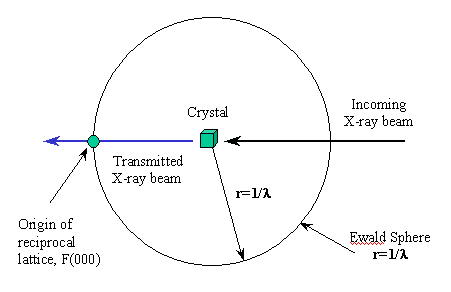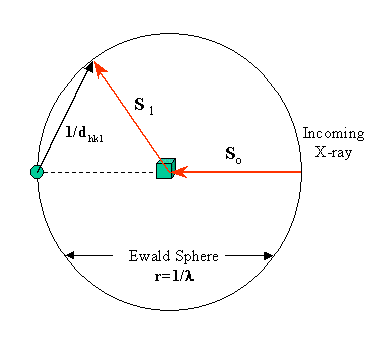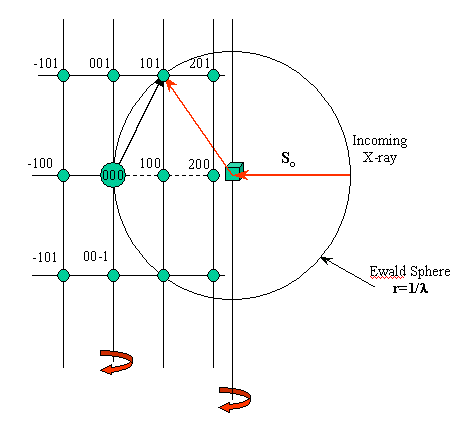#The Ewald Construction

### Intensity of diffraction spots

The observed intensity I of the diffraction spots can be thought of as corresponding to the 'size' of the reciprocal lattice point ( I(hkl) is proportional to |F(hkl)|2). Clearly, either depends on the contents of the unit cell, and we already suspect that the space group symmetry will thus have some implications on the diffraction pattern symmetry. Before we investigate further, it may be useful to understand how the diffraction pattern can be derived from the reciprocal lattice (RL). Let us look at a RL with spots in it :Here we see different magnitudes for the lattice points. The largest spot is at the origin corresponding to F(000), which we know already is the sum of all electrons in the unit cell. The reflection itself is at zero diffraction angle, i.e., in the primary beam path and not observable. Now, where do we expect all the other diffraction spots to appear?

### Ewald construction

A most useful means to understand the occurrence of diffraction spots is the Ewald construction. Let's begin slowly: We draw a sphere of radius 1/lambda, in the center of which we imagine the real crystal. The origin of the reciprocal lattice (RL, see above) lies in the transmitted beam, at the edge of the Ewald sphere.We know already that diffraction maxima (reflections, diffraction spots) occur only when the 3 Laue equations, or equivalent, the Bragg equation in vector form, are satisfied. This condition occurs whenever a reciprocal lattice point lies exactly on the Ewald sphere.As you may have assumed already, the chance for this to occur is modest, and we need to rotate the crystal in order to move more reciprocal lattice points through the Ewald sphere. In the following, I have drawn a reciprocal lattice in the origin, and we rotate it along the vertical axis of the drawing. We actually accomplish this by rotating the crystal along the same axis.Just imagine turning the RL through the Ewald sphere : in the beginning, only (101) and (10-1) give rise to a reflection. After you turned the RL a bit (which actually means turning the crystal around the same axis), the reciprocal lattice point 201 will enter the sphere and create a diffraction spot. Here are a few quiz questions to evaluate your understanding of this picture :

QUIZ

• Which direction are the reciprocal axes in this orthonormal RL pointed (a*,b*,c*)?

• What are the indices of the 2 unindexed green lattice points?

• Where would all the reflections be located if in the picture above you would put a cylindrical film (axis equal to rotation axis of crystal) around the crystal?

• In the given scenario, could there be a problem collecting data for, let's say 001, 002, 102 ?

• What would be the maximum d(hkl) (and hence, resolution) we can observe using a given wavelength?

Based on what we have learned so far, we will be able to construct a diffractometer and to begin data collection !Back to X-ray Tutorial Index
This World Wide Web site conceived and maintained by Bernhard Rupp.
Last revised Dezember 27, 2009 01:40